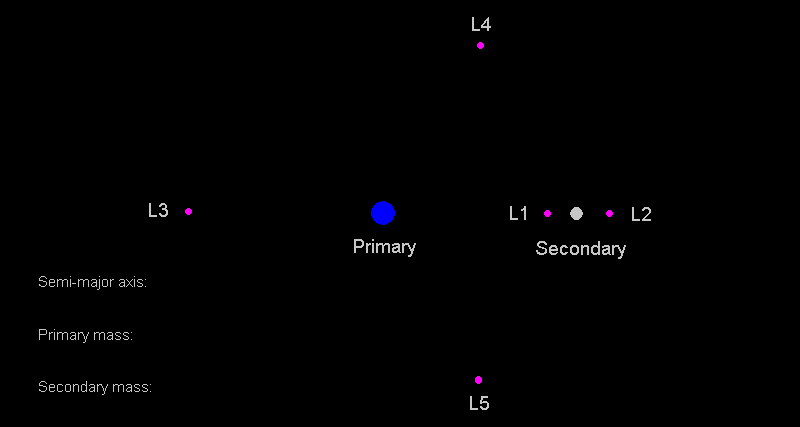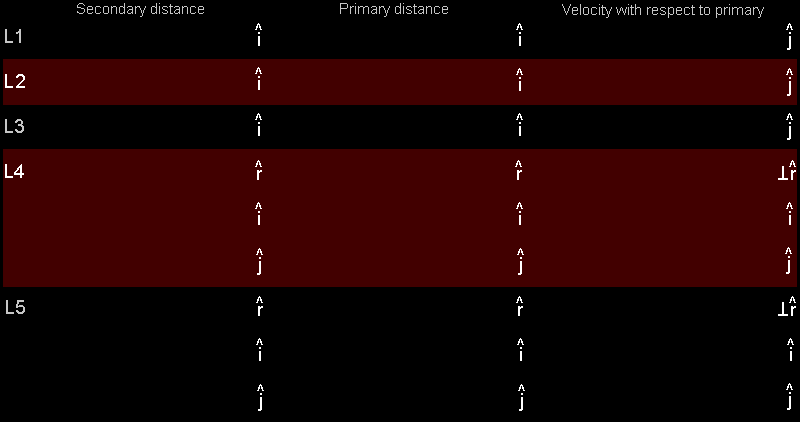This calculator computes the distance to L1, the distance to L2, the distance to L3, the distance to L4 and the distance to L5 for any two-body system. It assumes orbits are circular. It also computes the velocity necessary for an object placed on a Lagrange point to remain on the Lagrange point. In the cases of L1, L2, and L3, an object placed there would not be stable over long periods of time so the result is limited by the precision of Javascript's variables. Objects placed on L4 and L5, when given the proper velocity will remain there indefinately as long as the primary object is at least about 25 times as massive as the secondary object.

To use this calculator, simply enter the masses of the primary and secondary objects and the semi-major axis of the secondary's orbit around the primary. The calculator will automatically perform the computations. (c) 2008 by Tony DunnMore formulas